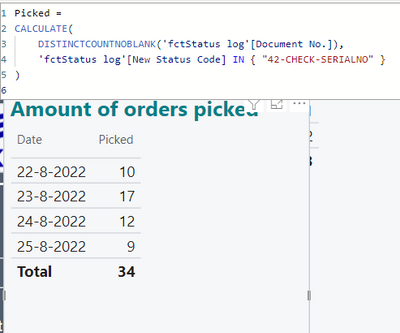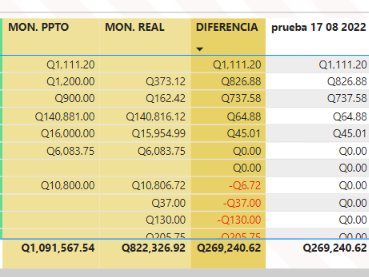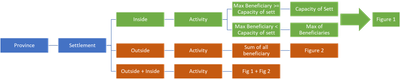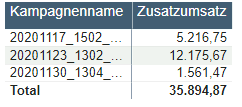cancel
Showing results for
Did you mean:Super User

## Dealing with Measure Totals

This one has come up quite a bit recently. The issue surrounds using Measures in Table visualizations with a Total row. The complaint is that the "Total" row is "wrong" for the measure. Technically, the total row is correct for the measure, it's just not what most people expect. What people expect is for the "Total" to display the sum of the values in the column. Measures do not do this. Measures respect the context of the Total row and is calculated within that context. Therefore, a Measure used in a column in a table visualization will likely have an unexpected value in the Total column.

There are a couple ways of fixing this. The easiest is to turn off the Total row.

Assuming that is not what you want, you can use the HASONEFILTER function to get around this issue. However, the ultimate solution will depend on how your measure is calculated.

For example, given the following data:

Year Amount

 Year1 500 Year2 1500 Year3 2000 Year4 100 Year5 800

We wish to find the total extra Amount spent above 1000 for each year. If the amount is not over 1000, we wish to display 0. To this end, we create a measure:

`MyMeasure = IF(SUM(Table[Amount])<1000,0,SUM(Table[Amount])-1000) `

Adding this to a Table visualization along with Year, we get the correct answer for each of the rows, but the Total line displays 3900, not 1500 as we would expect. The figure 3900 is calculated because the Measure is performing its calculation for ALL of the rows in the table, so the calculation is (500 + 1500 + 2000 + 100 + 800) - 1000 = 3900.

Correct, but not what was expected.

To get around this problem, use HASONEFILTER to calculate the Measure one way within a row context and another way within the Total row context, such as:

`MyMeasure2 = IF(HASONEFILTER(Table[Year]),IF(SUM(Table[Amount])<1000,0,SUM(Table[Amount])-1000),SUMX(FILTER(Table,[Amount]>1000),[Amount]-1000))`

Breaking this down, we essentially wrap our original measure in an IF statement that has the HASONEFILTER function as the logical test. If HASONEFILTER equals true, we calculate our Measure as before. However, if HASONEFILTER is false, we know that we have a Total row and we calculate our Measure a different way.

Become an expert!: Enterprise DNA
External Tools: MSHGQM
Latest book!:
Mastering Power BI 2nd EditionDAX is easy, CALCULATE makes DAX hard...
98 REPLIES 98Responsive Resident

Thx for the input!  I'm from from an expert at putting together formula's.  Can you provide a sample given the formula i sent in my original message?

ThanksFrequent Visitor

Greg,

I'm brand new to Power BI and I'm attempting to apply your solution.  My row dax works fine but the total is not working.  Change SF is a measure of (Sum of All Area - Start SF) and I want to split that between two new measures (Move-In and Move-Out).Any help is appreciated.

Thanks,

TonyNew Member

Hi,

I am having some difficulties with linking two separate data tables with a CALCULATE(SUM) calculated column. I want to bring in the total production cost at item level using the calculated column, but then apply a formula on this to generate the cost per unit in stock.

I have tried to do this using a calculated measure but the Total of the Measure does not equate to the sum of all of my table row values.

Urgent help on this would be greatly appreciated 🙂

Regards,

RyanFrequent Visitor

Hi,

I've created a measure to calculate the distinct "Document No." when they have a certain value (FILTER ON: '42-CHECK-SERIALNO').

It could be that the same Document No. will have the value (42-...) on a different date.

The totals are corrrect in the matrix, but the subtotals will count the value even if the Document No. has already hit the value (42-CHECK-SERIALNO) already on a different date. Herefor if you count the subtotals, you will get a different total als the one that is listed correctly in the matrix.

If the "Document No." occurs several times on different dates by value (42-...), then I do not want to count this (double) in the subtotals. It should only count the first time the "Document No." hits the status (42-...)I hope someone can help me with this issueSuper User

Hi,

What is the problem there.  The subtotal shows 34.  Are you expecting a different result there?

Regards,
Ashish Mathur
http://www.ashishmathur.comFrequent Visitor

Hi Ashish,

As you can see, the total is 34 which is correct. If you add up the subtotals (10+17+12+9 = 48), you get a different result. The addition of the subtotals is incorrect The subtotals will count the value even if the same Document No. has the value (42-CHECK-SERIALNO) on a different date.

Therefor, I would like to create a measure: If the "Document No." occurs several times on different dates by value (42-...), then I do not want to count this (double) in the subtotals. It should only count the first time the "Document No." hits the status (42-...).

Unfortunately, I cannot figure out how to do this.

Do you have experience how to do this?

PatrickSuper User

Hi,

Regards,
Ashish Mathur
http://www.ashishmathur.comAnonymous
Not applicable

It is shocking that after a number of years Power BI still does not offer an option to show the sum of the above columns as the total. Outside of a table - e.g. KPI - this "solution" breaks. This is disappointing.New Member

100% agree. Ridiculous that a BI tool would behave this way.Helper II

I'm totally agréé with you. In a visual table or others, the total row should have to be correct anyway the formula measure. Like in Excel with pivot table for instance. I would like PBI to correct this point to focus my reflection on the row ans not on the total.Frequent Visitor

.Regular Visitor

I am lookin at this thread , to solve a similar problem and I tried the Sumx soln as well has HASONEVALUE but overall sum I am still not able to sum up to expected. Can you please Advise what wrong with the my formula .

Source Data(Calculation in Excel)  :

 Distri EAN Local Actual Forecast MAE Weight MAPE x Weight WMAPE BD 47400115507 19.25 17.56442 9% 3.1779% 0.28% 16% BD 47400179240 116.0995 122.743 6% 19.1665% 1.10% BD 47400179349 34.441 25.14863 27% 5.6858% 1.53% BD 3014260007836 2.0016 4.536926 127% 0.3304% 0.42% BD 3014260007867 4.3924 5.480001 25% 0.7251% 0.18% BD 3014260781033 1.3344 5.009387 275% 0.2203% 0.61% BD 4902430044233 32.46672 30.18235 7% 5.3598% 0.38% BD 4902430044257 26.0003 17.23862 34% 4.2923% 1.45% BD 4902430102247 227.6804 247.6915 9% 37.5871% 3.30% BD 4902430102254 100.3049 128.6972 28% 16.5591% 4.69% BD 4902430118651 16.10251 12.51005 22% 2.6583% 0.59% BD 4902430188937 13.66653 20.08869 47% 2.2562% 1.06% BD 4902430188951 12 8.71067 27% 1.9810% 0.54% 16%

Ouput in PowerBI :Using SUMX:

MAPE * Weight =
VAR _MAE = CALCULATE(IF(temp_ean[Measure_Actual]=0,1,ABS(temp_ean[Measure_Actual]-temp_ean[Measure_Forecast])/temp_ean[Measure_Actual]))
VAR _WEIGHT = DIVIDE(temp_ean[Measure_Actual] , temp_ean[TotalActual])
RETURN

SUMX(VALUES(temp_ean[Distro]) , _MAE * _WEIGHT)

Using HASONEVALUE:
Measure1 =
VAR __table = SUMMARIZE(temp_ean,temp_ean[Distro],"__value",temp_ean[MAPE * Weight])
RETURN
IF (
HASONEVALUE(temp_ean[Distro]),
temp_ean[MAPE * Weight],
SUMX(__table,[__value])
)

Any help is appreciated!

ThanksSuper User

Hi,

Regards,
Ashish Mathur
http://www.ashishmathur.comFrequent Visitor

Buen dia, me considero nuevo en esto,

si me puede ayudar por favor, tengo la siguiente tablalos archivos estan relacionados entre si.

1.- el MON. PPTO lo obtengo de un archivo, de la columna de totales

2.- el MON. REAL lo obtengo de otro archivo, de la columna de totales

3.- la diferencia la obtengo de la resta entre estas dos columnas de diferentes archivos usando esta medida --

DIFERENCIA = SUM('PRESUPUESTO DIRECTO'[MON_TOTAL]) - SUM('COSTO DIRECTO'[MON_TOTAL])
(si se pudiera una solucion desde esta medida para que me sume solo los positivos seria excelente)

el problema es que quiero obtener una columna mas con la suma unicamente de los valores positivos, que es el dato de la columna sin color en la imagen, el resultado de la medida es el correcto pero no el esperado por mi persona, ya que la medida esta haciendo su funcion lineal.
PRESUPUESTO POR EJECUTAR = if(SUMX('PRESUPUESTO DIRECTO','PRESUPUESTO DIRECTO'[MON_TOTAL]) > sum('COSTO DIRECTO'[MON_TOTAL]),SUMX('PRESUPUESTO DIRECTO','PRESUPUESTO DIRECTO'[MON_TOTAL]) - SUM('COSTO DIRECTO'[MON_TOTAL]))

ME PODRIA AYUDAR DANDOME UNA SOLUCION PARA QUE EL TOTAL ME SUME UNICAMENTE LOS VALORES POSITIVOS, YA REALICE VARIAS MEDIDAS PERO NINGUNA ME DA EL RESULTADO ESPERADO,  ESPERO DARME A ENTERDERFrequent Visitor

@Greg_Deckler  Hasonevalue is working if we are dealing with Existing Colomn I have tried on top of my measure its not working as x function we can not use in case of masure.

My problam is:-

1) we have two masure in table visualization with total like masure A & B

2) requirement is if (A-B<0,0,A-B), so i need to takeout the all +ve value from A-B(and assign -ve and 0 as 0), Then the total should be there,I am able to achive The above but Total is wrong (when i am adding all values indivually total is different which is actual )

using following masure----

masure C= A-B, Total = SUMX(FILTER(ALLSELECTED(Table[column]),[C]>0),[C]) note-: Working wrong at Total level

here Table[column] is ref. coloum for row filter... ThanksFrequent Visitor

it works for me after doing some R&D.

measure C = meaasure A- Measure B.

+ve total = Calculate([C],FILTER(SUMMARIZE(FACTTABLE,PARENTDIMTABLE[COLUMN],FACTTABLE[COLUMN]),[C]>0))Regular Visitor

I want to calculate thisAnd created the following measure -

But says somewhere is wrong, but can't find it 😞

IF(SUM ( 'MasterTbl'[Sett] ) = 1,

SUMX (
(IF (
SUM ( 'MasterTbl'[Sett] ) = 0,
CALCULATE (
SUM ( 'MasterTbl'[Total number of beneficiaries] ),
ALLEXCEPT ( MasterTbl, 'MasterTbl'[Activities and Indicators.Activities] )
) )),

IF

(
VAR _categoryfirst =
CALCULATETABLE (
VALUES ( 'MasterTbl'[List of refugee settlements-Iran.settlements name] ),
"MaxValue",
CALCULATE (
MAX ( 'MasterTbl'[Total number of beneficiaries] ),
ALL ( 'MasterTbl'[Total number of beneficiaries] )
)
),
ALL ( 'MasterTbl'[Total number of beneficiaries] )
)
RETURN
CALCULATE (
MAX ( 'MasterTbl'[Total number of beneficiaries] ),
KEEPFILTERS (
TREATAS (
_categoryfirst,
'MasterTbl'[List of refugee settlements-Iran.settlements name],
'MasterTbl'[Total number of beneficiaries]
)) )) >= SUM('Settlement name'[population]), SUM('Settlement name'[population])),
(
VAR _categoryfirst =
CALCULATETABLE (
VALUES ( 'MasterTbl'[List of refugee settlements-Iran.settlements name] ),
"MaxValue",
CALCULATE (
MAX ( 'MasterTbl'[Total number of beneficiaries] ),
ALL ( 'MasterTbl'[Total number of beneficiaries] )
)
),
ALL ( 'MasterTbl'[Total number of beneficiaries] )
)
RETURN
CALCULATE (
MAX ( 'MasterTbl'[Total number of beneficiaries] ),
KEEPFILTERS (
TREATAS (
_categoryfirst,
'MasterTbl'[List of refugee settlements-Iran.settlements name],
'MasterTbl'[Total number of beneficiaries]
) ) ) ) ),

(   IF (
SUM ( 'MasterTbl'[Sett] ) = 0,
CALCULATE (
SUM ( 'MasterTbl'[Total number of beneficiaries] ),
ALLEXCEPT ( MasterTbl, 'MasterTbl'[Activities and Indicators.Activities] )
),
IF
(
(
VAR _categoryfirst =
CALCULATETABLE (
VALUES ( 'MasterTbl'[List of refugee settlements-Iran.settlements name] ),
"MaxValue",
CALCULATE (
MAX ( 'MasterTbl'[Total number of beneficiaries] ),
ALL ( 'MasterTbl'[Total number of beneficiaries] )
)
),
ALL ( 'MasterTbl'[Total number of beneficiaries] )
)
RETURN
CALCULATE (
MAX ( 'MasterTbl'[Total number of beneficiaries] ),
KEEPFILTERS (
TREATAS (
_categoryfirst,
'MasterTbl'[List of refugee settlements-Iran.settlements name],
'MasterTbl'[Total number of beneficiaries]
)
)
)
) >= SUM('Settlement name'[population]), SUM('Settlement name'[population]),
(
VAR _categoryfirst =
CALCULATETABLE (
VALUES ( 'MasterTbl'[List of refugee settlements-Iran.settlements name] ),
"MaxValue",
CALCULATE (
MAX ( 'MasterTbl'[Total number of beneficiaries] ),
ALL ( 'MasterTbl'[Total number of beneficiaries] )
)
),
ALL ( 'MasterTbl'[Total number of beneficiaries] )
)
RETURN
CALCULATE (
MAX ( 'MasterTbl'[Total number of beneficiaries] ),
KEEPFILTERS (
TREATAS (
_categoryfirst,
'MasterTbl'[List of refugee settlements-Iran.settlements name],
'MasterTbl'[Total number of beneficiaries]
) )  ) ) )  )))Helper II

Hi Greg,
I realise this is an old post but it seems to still be, very much, relevent as I know loads of people who struggle with this concept.

I've tried to replicate the method you use for my own problem and kind of get half way but then hit the gravel.
Is there any chance you could apply your process to the issue I posted?
https://community.powerbi.com/t5/Desktop/Totals-Calculations-using-a-a-real-problem/m-p/2250407

Thanks

PaulHelper I

Hi everyone,

I'm also having a problem with column totals with the following measure:

Zusatzumsatz =

VAR Zielgruppe =
CALCULATE(
[Umsatz pro Käufer],
FILTER('Table1''Table1'[CONTROLGROUP] = "Zielgruppe")
)

VAR Datum =
MAX(Table1[SENDDATE])

VAR ProgrammID =
MIN(Table1[Program ID])

VAR Kontrollgruppe =
CALCULATE(
[Umsatz pro Käufer],
Table2[PROGRAMID] = ProgrammID,
Table1[SENDDATE] = Datum,
Table1[CONTROLGROUP] = "Kontrollgruppe",
ALL()
)

VAR IstZielgruppe =
MAX(Table1[CONTROLGROUP])="Zielgruppe"

VAR Auflage_Final =
CALCULATE( SUM(Table1[AUFLAGE]), KEEPFILTERS(Table1[CONTROLGROUP]="Zielgruppe"))

RETURN
IF(IstZielgruppe,
(Zielgruppe - Kontrollgruppe)* Auflage_Final
)As you can see - the column total is wring. I understand that the measure is calculating in row and not column context. Is there a way that the calculation in the value part of the table is evaluated per row but the total is just the sum of the column?Super User

Hi,

Drag this measure to your visual

Measure = SUMX(VALUES(Data[kampagnenname]),[Zusatzumsatz])

Hope this helps.

Regards,
Ashish Mathur
http://www.ashishmathur.com